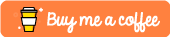#Welcome to blog of Arun Gopinath

# Introduction

In this tutorial lets us learn how to extract the boundary limits or bbox values of each polygon in a shapefile using QGIS. First of all, imagine a shapefile consists of some number of polygons. So each polygon can be exactly fit into imaginary rectangles each having upper and lower limits of latitude and longitude values. These four limits will be the boundary for that polygon. Similarly, remaining polygons will also have such boundaries. In this article, we will extract those values into a spreadsheet format.

## Required tools

• QGIS

• Mircrosoft Excel

## Follow these steps

To calculate the boundary extend of a shapefile ( Contains multiple polygons )

1. Open the required shapefile in QGIS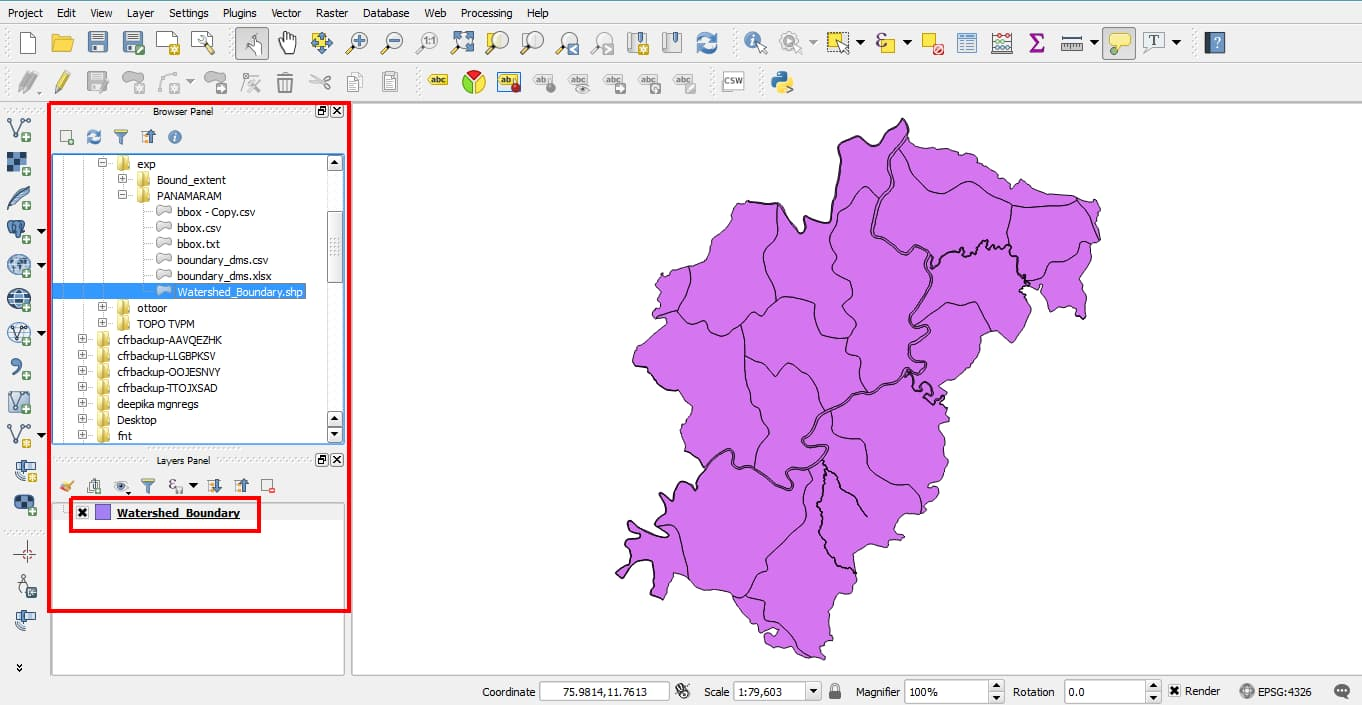1. Now open attribute table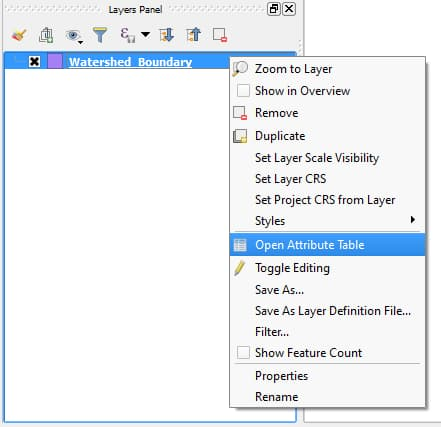1. Click Start editing button (pencil) [Ctrl + E].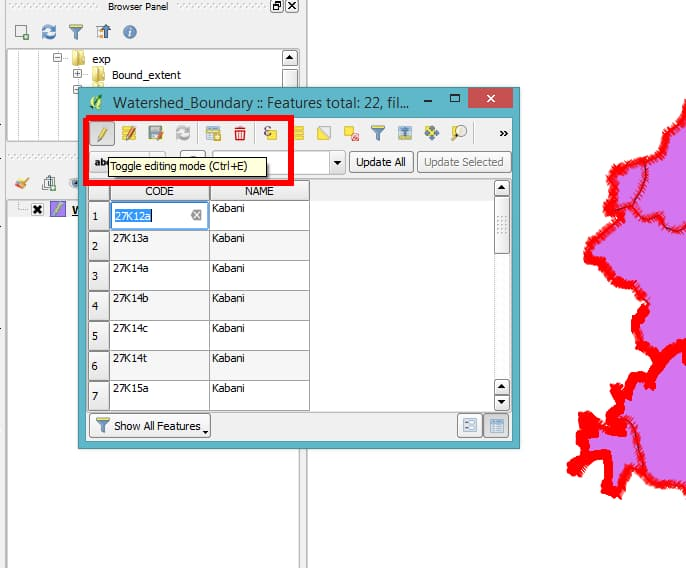Also, select ‘Open Field Calculator’ [ Ctrl + I ]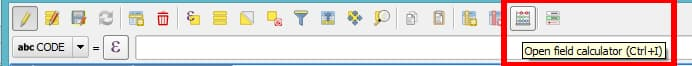1. Check create new field, Add output field name as xmin

xmin and xmax represents North(upper limit) and South (lower limt) of boundary respectively. Similarly ymin and ymax represents West and East boundary limts.

1. Output field type as ‘text’

2. Output field type = 20 (Optional)

3. In the Expression tab below, enter

x_min($geometry)  If everything is fine, then an output preview can be seen in the left bottom of that tab.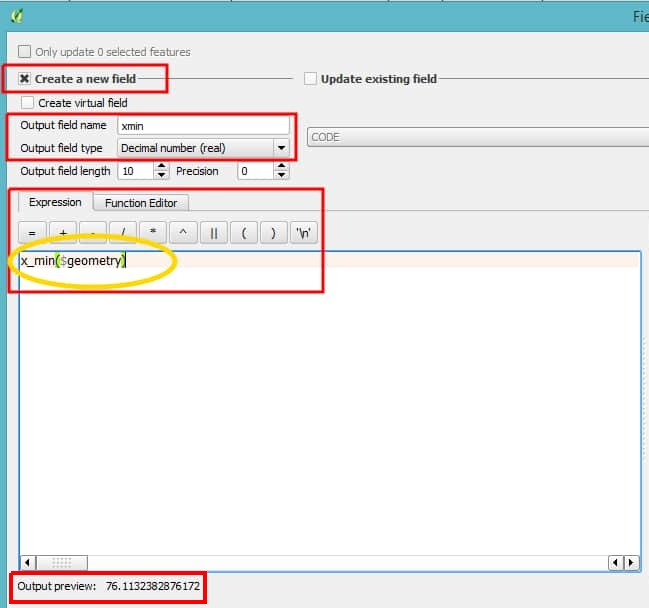1. Click OK. The attribute table will be populated with xmin values.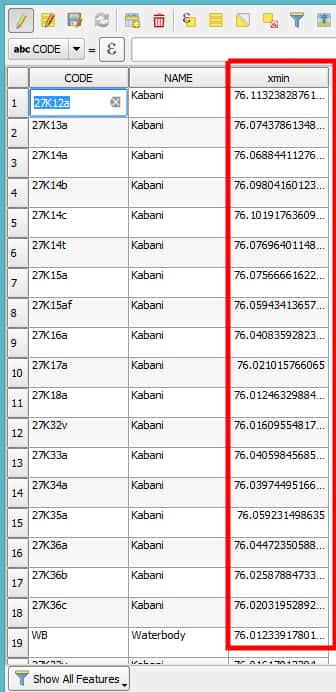The values will be in decimals. We have to convert it into DMS(Degree,Minute,Seconds) later. • Similarly, follow the above steps for xmax, ymin & ymax. Respective expressions will be x_max($geometry)

y_min($geometry) y_max($geometry)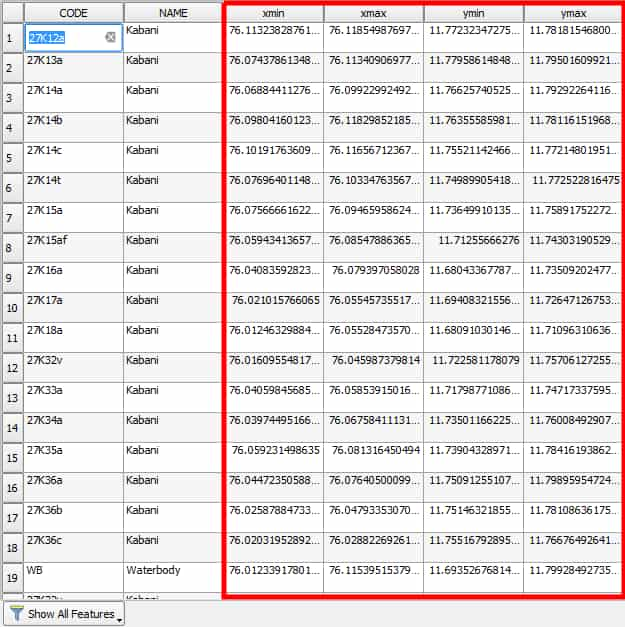1. Now in the layers panel of QGIS, right click to save as the shape file as .csv format and click save.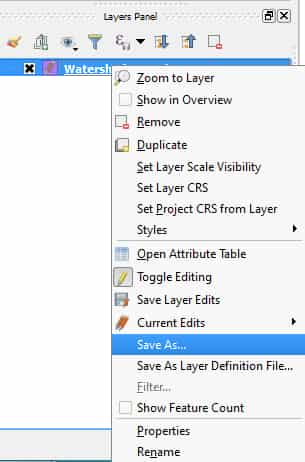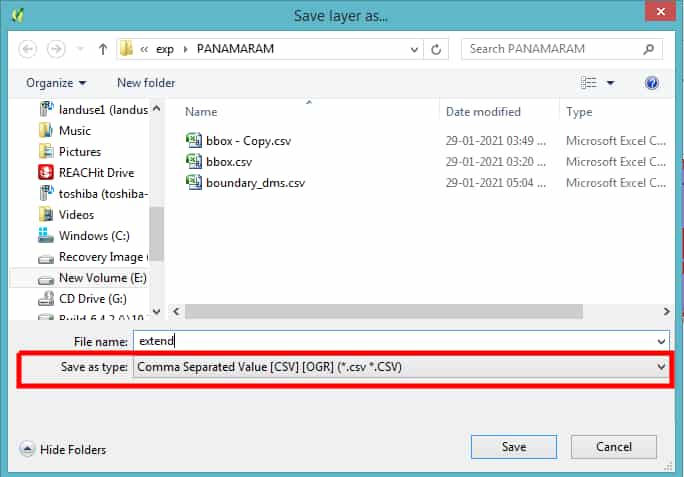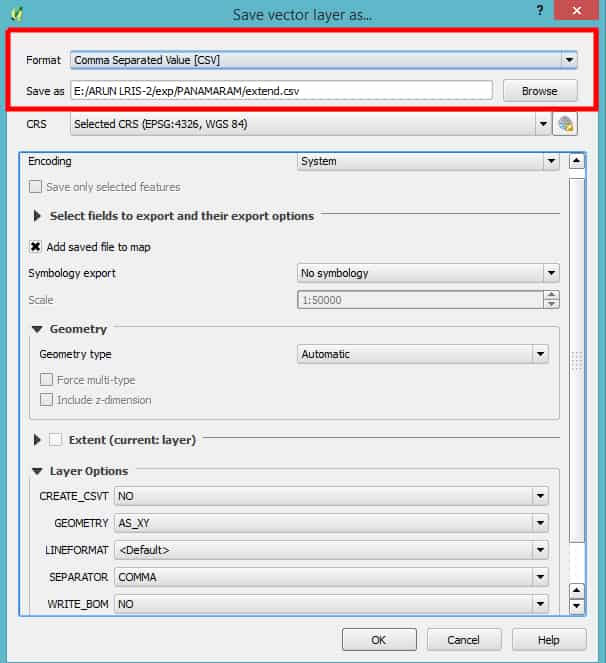1. Now open the above saved spread sheet in Excel.

2. Add XMIN, XMAX, YMIN and YMAX columns.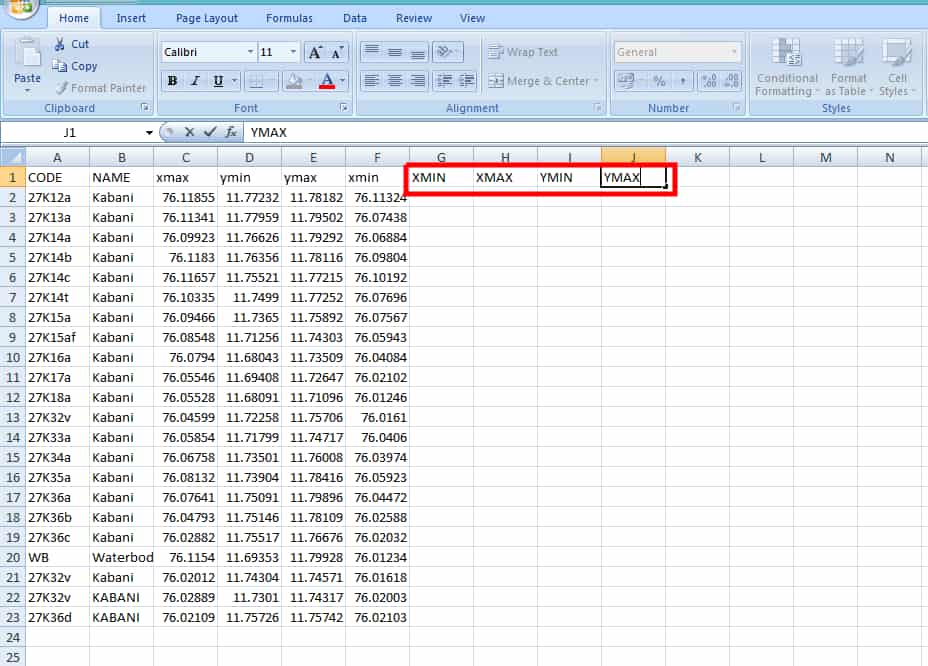1. Click Alt + F11 , which means you are opening the Visual Basic for Applications (VBA)

2. Select Insert , then Module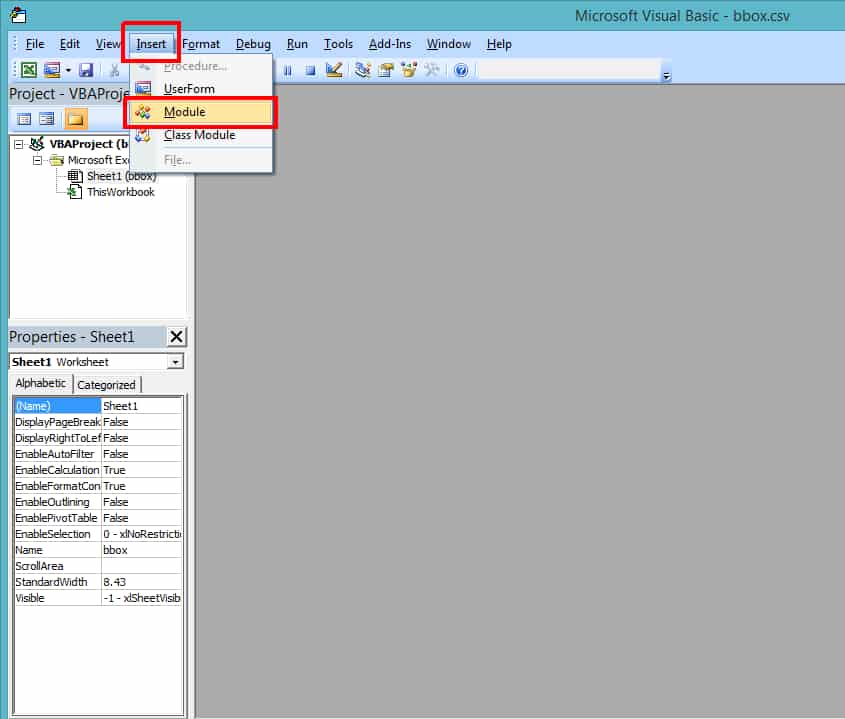1. A blank tab will open up. Copy the code given below and paste it in that blank space.
Function Convert_Degree(Decimal_Deg) As Variant
With Application
'Set degree to Integer of Argument Passed
Degrees = Int(Decimal_Deg)
'Set minutes to 60 times the number to the right
'of the decimal for the variable Decimal_Deg
Minutes = (Decimal_Deg - Degrees) * 60
'Set seconds to 60 times the number to the right of the
'decimal for the variable Minute
Seconds = Format(((Minutes - Int(Minutes)) * 60), "0.000")
'Returns the Result of degree conversion
'(for example, 10.46 = 10~ 27  ' 36")
Convert_Degree = " " & Degrees & "° " & Int(Minutes) & " ' " & Seconds + Chr(34)
End With
End Function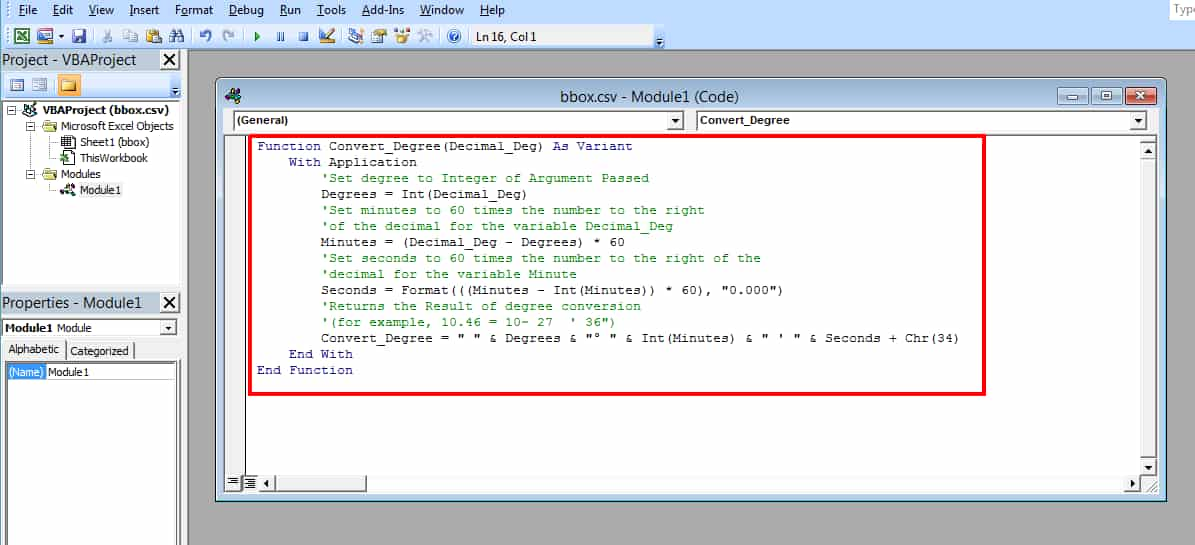Click Alt + F11 to go back to the spreadsheet.

1. Now select the cell just below the XMIN and type
=Convert_Degree(C2)


in the formula box and click ENTER.

Change the C2 with the cell name contains the first decimal value of xmin.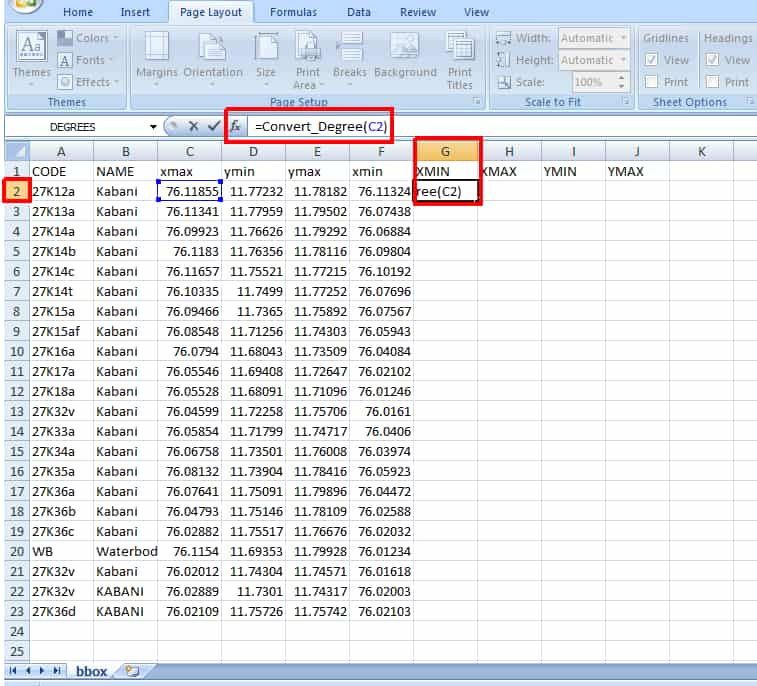1. Drag the + sign in the right bottom corner of the same cell to the last valued row.

2. Repeat the same for XMAX, YMIN and YMAX.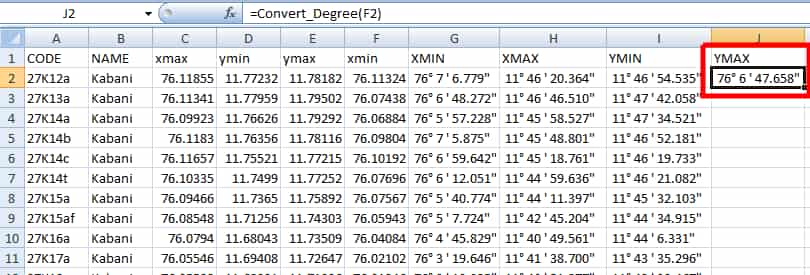Note: Remember to change the cell value in the Step 15 in next steps.

1. Save the file in Excel macro enabled format (.xlxm). By selecting this format, the module we added in VBA will be attached with the workbook.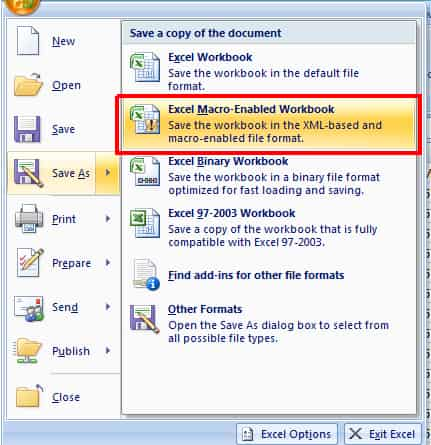### Things to be noted

If the required output is in decimal values, then up to step 9 is enough. Those processes are also possible in ArcGIS software.